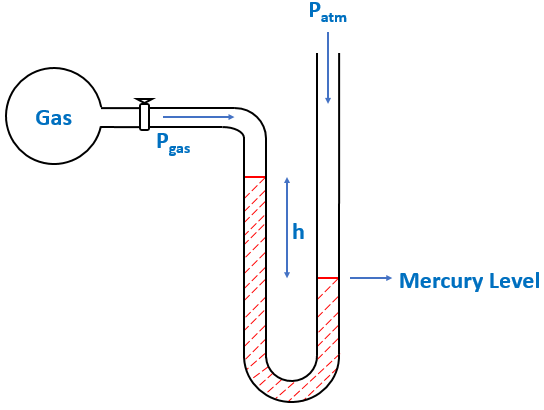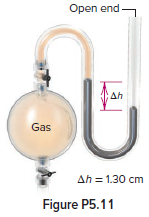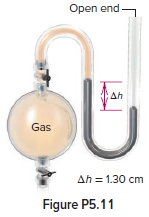# Problem: In Figure P5.11, what is the pressure of the gas in the flask (in kPa) if the barometer reads 765.2 mmHg?

🤓 Based on our data, we think this question is relevant for Professor Waddell's class at UC.

###### FREE Expert Solution

When Patm is pushing harder than  Pgas$\overline{){{\mathbf{P}}}_{\mathbf{g}\mathbf{a}\mathbf{s}}{\mathbf{=}}{{\mathbf{P}}}_{\mathbf{a}\mathbf{t}\mathbf{m}}{\mathbf{-}}{\mathbf{h}}}$$\overline{){{\mathbf{P}}}_{\mathbf{g}\mathbf{a}\mathbf{s}}{\mathbf{=}}{{\mathbf{P}}}_{\mathbf{a}\mathbf{t}\mathbf{m}}{\mathbf{-}}{\mathbf{h}}}$

Given:

Patm = 765.2 mmHg
h = 1.30 cm

Convert h first from cm to mm:###### Problem Details

In Figure P5.11, what is the pressure of the gas in the flask (in kPa) if the barometer reads 765.2 mmHg?